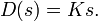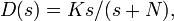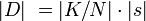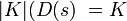# FAQ: How does a pole at high frequency prevent PID amplification of high frequency noise?

Jump to: navigation, search

Posted by Shreesh Mysore, 25 November 2002
To understand this recall that high frequencynopise amplification is caused mainly by the "D" part of the PID controller. Consider the transfer function due to the D part (lets call it D(s)):We see that as gets large, so does <mat>|D|[/itex]. To offset this effect, what we need is a means to limit high frequency gain, i.e., to bound the value of. Let us consider a modified version of D(s) aswith N being large. The interpretation is that we have "added" a high frequency pole to the transfer function.

So how does this help? At low frequencies (small s), we see that(because N is chosen large). Therefore,. Thus at low frequencies, the transfer function behaves just like the plain differentiatior (Ks) - our old transfer function. At high frequencies (s large), we see that s swamps out N, and hence. The high frequency gain is therefore limited by.